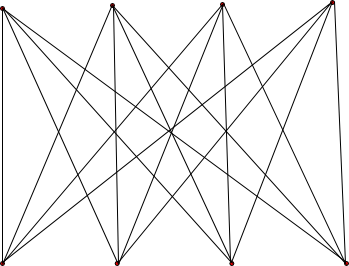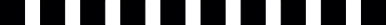## CS 124, Fall 2009 Solutions to Test #1

1. Explain the terms machine language and high-level language, and discuss the relationship between them. What does this have to do with the javac command?

Answer: Machine language and high-level language are both languages in which computer programs can be written. In machine language, instructions are coded into binary numbers that are executed directly by the CPU of the computer. Machine language is not meant to be read by or written by humans. In a high-level language such as Java, programs are written in a form that is closer to ordinary human language (although still very limited and strict). High-level language programs are written by people, but before they can be executed by the computer, they must be translated into machine language. The javac command is used to translate Java programs into machine language -- although in this case, it is machine language for the "Java Virtual Machine" rather than real, physical computer. Jave Virtual Machine language still has to be interpreted (by the java command) in order to run it on a real computer.

2. Explain the terms syntax and semantics, and illustrate your explanation with some examples of how the terms apply to Java.

Answer: Syntax refers to the grammar of a language. That is, it is the set of rules that determine what is and is not legal in the language. Semantics refers to the meaning of a language. That is, it is the set of rules that determine what phrases in the language mean.

In the case of Java, a syntactically correct program is one that can be successfully compiled. A semantically correct program is one that will give the correct result when it is run.

Looking at a statement such as "x = x + 1;", we say that this follows the syntax of an assignment statement: It consists of a variable followed by "=" followed by an expression. The semantics of this statement is what it does; that is, it adds 1 to the value stored in the variable x.

3. In programming, style rules are important, even though the computer ignores them. Explain why this is true, and discuss a few of the style rules that a good programmer will follow when writing Java programs.

Answer: Style rules are ignored by the computer, but they are useful for human readers of the program. Good style can make it easier to read and understand the program, and it can make it easier to locate errors while debugging. Style is important because the audience for a program includes people, not just machines.

Some style rules in Java include the use of comments and indentation. Comments can help the reader understand what the program is trying to do. Indentation lets the user see the structure of the program more easily. Another style rules is that variables should have meaningful names, and that their names should begin with lower case letters.

4. Define the term literal as it relates to computer programs, and give two examples of literals of different types.

Answer: A literal is simply a notation that is used in a program to represent a constant value. For example, the literal 17 represents the numeric value 17, and the literal 'A' is a literal of type char that represents the upper-case letter A. (Note that the quote marks in 'A' are part of the literal, but are not part of the value that is represented.)

5. Describe all possible outputs from the following code segment, and give an example of one possible output.

```		int n;
double x;
x = 5 * Math.random();
n = (int)(x);
System.out.printf("\%d \%1.2f", n, x);
```

Answer: The value of x will be a real number that satisfies 0 <= x < 5. Since x cannot be equal to 5, when it is rounded down to an integer with (int)(x), the result is strictly less than 5. So, n is one of the integers 0, 1, 2, 3, or 4. When n and x are printed out with the formatted print statement, the result is an integer, followed by a space, followed by a real number written with two digits after the decimal place. For example:

```            4 4.37
```

The integer can be anything from 0 to 4, while the real number can be anything from 0.00 to 5.00. Note that the output can be 5.00, even though x is strictly less than 5.00. This is because the output will be rounded to two decimal places. Thus, for example, if x is 4.999, then the rounded output will be 5.00. Similarly, you can get the output "3 4.00" when x is, for example, equal to 3.9975.

6. Show the output that would be produced when the following code segment is executed:

```	   int n, x, y;
x = 1;
y = 5;
while ( x <= y ) {
x = x*3;
y = y*2;
n = y / x;
System.out.println(n + " " + x + " " + y);
}
```

Answer: This problem should be done by blindly tracing the values of n, x, and y as the code segment is executed. Here is the output:

```             3 3 10
2 9 20
1 27 40
0 81 80
```

7. Draw the picture that would be produced by the following paintComponent() method:

```      protected void paintComponent(Graphics g) {
int i, j;
for (i = 0; i <= 3; i++) {
for (j = 0; j <= 3; j++) {
g.drawline( 100*i, 0, 100*j, 300);
}
}
}
```

Answer: The for loops in this code segment are nested. The outer loop is executed four times. For each execution of the outer loop, the inner loop is executed four times. The statement inside the inner loop is therefore executed 16 times. A total of 16 lines are drawn. These lines connect each of the points (0,0), (100,0), (200,0), (300,0) to each of the points (0,300), (100,300), (200,300), and (300,300). Here is the picture:8. Suppose that the price of admission to a museum depends on the customer's age. Children 12 years old or younger pay \$2.00. Senior citizens aged 62 or over pay \$3.00. General admission for everyone else is \$5.00. Write a program segment that will ask the user to enter his or her age, and then print a message stating the user's price of admission. (Use an if statement.) You don't have to write a complete program, but you should declare any variable that you use.

```          int age;
age = TextIO.getlnInt();
if ( age <= 12 ) {
}
else if ( age >= 62 ) {
}
else {
}
```

9. Write a code segment to simulate the following experiment: Toss a coin over and over, until it has come up heads ten times. At the end, print the number of times that the coin has come up tails.

```     int heads;
int tails;
tails = 0;
while ( heads < 10 ) {
if ( Math.random() < 0.5 ) {  // count this as a head
}
else {  // count this as a tail
tails++;
}
}
// At this point, we know that heads == 10.
System.out.println("The number of tails was " + tails);
```

10. Write a paintComponent method that draws a horizontal line of ten squares, like the one shown here. Use a for loop and the g.fillRect() subroutine. The size and color of the boxes are not important.```        protected void paintComponent(Graphics g) {
g.setColor(Color.BLACK);
int i;
for (i = 0; i < 10; i++) {
g.fillRect(20*i,0,10,10);
}
}
or:
protected void paintComponent(Graphics g) {
g.setColor(Color.BLACK);
int x;  // The x-coordinate of one of the squares
for (x = 0; x < 200; x = x + 20) {
g.fillRect(x,0,10,10);
}
}
```

11. Write a complete Java program (starting with public class...) to do the following. The program will get two real numbers from the user, representing the lengths of two sides of a right triangle. The program should compute the length of the hypotenuse of the triangle, and print out the result. (If the input numbers are a and b, then the hypotenuse is the square root of a squared plus b squared. This problem mostly tests your knowledge of the format of a Java program. The program is not meant to be sophisticated.)

```       public static class Pythagorus {

public static void main(String[] args) {
double x, y; // The sides of the triangle, input from the user.
double hypotenuse;
System.out.print("Enter the first side length:   ");
x = TextIO.getlnDouble();
System.out.print("Enter the second side length:  ");
y = TextIO.getlnDouble();
hypotenuse = Math.sqrt( x*x + y*y );
System.out.printf("The hypotenuse is %1.6g.\n", hypotenuse);
}

}
```

12. One of the central ideas in computer science is algorithm. Explain what is meant by an algorithm and what it has to do with computer programs. Also discuss how pseudocode and stepwise refinement can be used by a programmer to help develop an algorithm.

Answer: An algorithm is a step-by-step procedure for carrying out some task, which is guaranteed to terminate after a finite number of steps.

A computer program is an expression of an algorithm. An algorithm that has been expressed as a computer program can be carried out mechanically by a computer. But there are other ways of expressing algorithms, such as in plain English. English, however, tends to be ambiguous, and it is often hard to express algorithms precisely in English. Another option is pseudocode. Pseudocode is similar to a programming language, but it is more informal, and sometimes it just describes complex steps instead of spelling them out.

Pseudocode can be useful when developing algorithms. The developer can start with a broad outline of the algorithm, expressed in pseudocode. That algorithm can be be refined by spelling out complex steps in more detail. After enough detail has been added, it will become possible to translate the algorithm into an actual programming language.